# Equation with abs value

How many solutions has the equation
$\left(\mathrm{\mid }x\mathrm{\mid }+x\right)\mathrm{\mid }x-3\mathrm{\mid }=\mathrm{\mid }x+1\mathrm{\mid }$
in the real numbers?

n =  4

### Step-by-step explanation:

The equation has infinity many solutions.

Our simple equation calculator calculates it.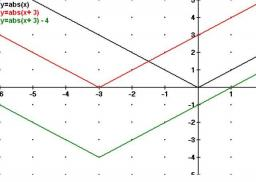Did you find an error or inaccuracy? Feel free to write us. Thank you!Tips to related online calculators
Looking for help with calculating roots of a quadratic equation?
Do you have a linear equation or system of equations and looking for its solution? Or do you have a quadratic equation?

## Related math problems and questions:

• Equation with absSolve this equation with absolute value member: |3(3x-1)|=8
• RootsDetermine the quadratic equation absolute coefficient q, that the equation has a real double root and the root x calculate: ?Determine the numbers b, c that the numbers x1 = -1 and x2 = 3 were roots of quadratic equation: -3x 2 + b x + c = 0
• ABS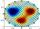What is the value of ? ?
• SupposeSuppose you know that the length of a line segment is 15, x2=6, y2=14 and x1= -3. Find the possible value of y1. Is there more than one possible answer? Why or why not?
• Factors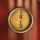Can the expression ? be factored into rational factors?
• ABS CNCalculate the absolute value of complex number -15-29i.
• Domains of functions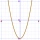F(x)=x2-7x and g(x)=5-x2 Domain of (fg)(x) is. .. . . Domain of (f/g)(x). ..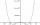By introducing a new variable solve biquadratic equation: - x 4 +277 x2 -15876=0Write the equation of the quadratic function, which includes points A (-1, 10), B (2, 19), C (1,4)
• Non linear eqsSolve the system of non-linear equations: 3x2-3x-y=-2 -6x2-x-y=-7
• SquarePoints A[9,9] and B[-4,1] are adjacent vertices of the square ABCD. Calculate the area of the square ABCD.
• Vectors abs sum diff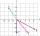The vectors a = (4,2), b = (- 2,1) are given. Calculate: a) |a+b|, b) |a|+|b|, c) |a-b|, d) |a|-|b|.
• Equation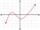Eequation f(x) = 0 has roots x1 = 64, x2 = 100, x3 = 25, x4 = 49. How many roots have equation f(x2) = 0 ?
• CylindersThe area of the side of two cylinders is the same rectangle of 33 mm × 18 mm. Which cylinder has a larger volume and by how much?Quadratic equation 7x2+bx+c=0 has roots x1 = 67 and x2 = -84. Calculate the coefficients b and c.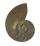Solve equation: log13(7x + 12) = 0